# 3.9: Compound Inequalities

•• Contributed by CK12
• CK12

## Compound Inequalities

Suppose you spent 2 more hours doing your homework this week than you spent last week. If the number of hours that you spent last week is represented by h, and you spent between 6 and 9 hours this week, how many hours did you spend doing your homework last week?

### Compound Inequalities

Inequalities that relate to the same topic can be written as a compound inequality. A compound inequality involves the connecting words “and” and “or.”

The word and in mathematics means the intersection between sets or what the sets have in common.

The word or in mathematics means the union of the sets or combining both sets into one large set.

#### Inequalities Involving “And”

Suppose you were given the statement “The speed limit is 65 miles per hour.” Using interval notation, the solutions to this situation can be written as [0, 65]. As an inequality, what is being said it this:

The speed must be at least 0 mph and at most 65 mph.

Using inequalities to represent “at least” and “at most,” the following sentences are written:

s≥0 and s≤65

This is an example of a compound inequality. It can be shortened by writing:

0≤s≤65

#### Graph the solutions to −40≤y<60:

Color in a circle at -40 to represent “less than or equal to.” Draw an open circle at 60 to indicate that 60 is the limit, but is not itself included. The variable is placed between these two values, showing that the solutions occur between these two numbers.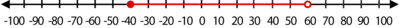#### Solving “And” Compound Inequalities

When you solve compound inequalities, separate the inequalities and solve each of them separately. Then combine the solutions at the end.

#### 3x−5<x+9≤5x+13

To solve this compound inequality, begin by separating the two component inequalities.

3x−5<x+9

2x<14

x<7

and

x+9≤5x+13

−4≤4x

−1≤x or x≥−1

The answers are x<7 and x≥−1 and can be written together as −1≤x<7. Graph the solutions that satisfy both inequalities.

#### Inequalities Involving “Or”

Suppose a restaurant offers discounts to diners who are children 3 years or younger or adults over 65.

To write an inequality to represent this situation, begin by writing an inequality to represent each piece. “3 years or younger” means you must be born but must not have celebrated your fourth birthday.

0≤a<4

To finish writing the inequality, add the word "or" between the phrases.

0≤a<4 or a>65

The word "or" between the phrases allows you to graph all the possibilities on one number line.

#### Solving “Or” Compound Inequalities

To solve an “or” compound inequality, separate the component inequalities, solve each separately, then combine the solutions to finish the problem.

#### Solve for x and graph the solution to the following inequality: 9−2x≤3 or 3x+10≤6−x

To solve this compound inequality, begin by separating the components.

9−2x≤3

−2x≤−6

x≥3

or

3x+10≤6−x

4x≤−4

x≤−1

The answer is x≥3 or x≤−1. Graph by placing filled dots at 3 and at -1, then coloring the line in opposite directions to indicate the values of x that make either statement true.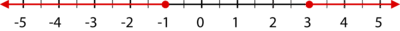#### Using a Graphing Calculator to Solve Compound Inequalities

Graphing calculators can be used to solve many complex algebraic sentences.

#### Solve 7x−2<10x+1<9x+5 using a graphing calculator:

This is a compound inequality composed of two separate statements: 7x−2<10x+1 and 10x+1<9x+5.

To enter a compound inequality into a graphing calculator:

Press the [Y=] button.

The inequality symbols are found by pressing [TEST] [2nd] [MATH]

Enter the inequality as: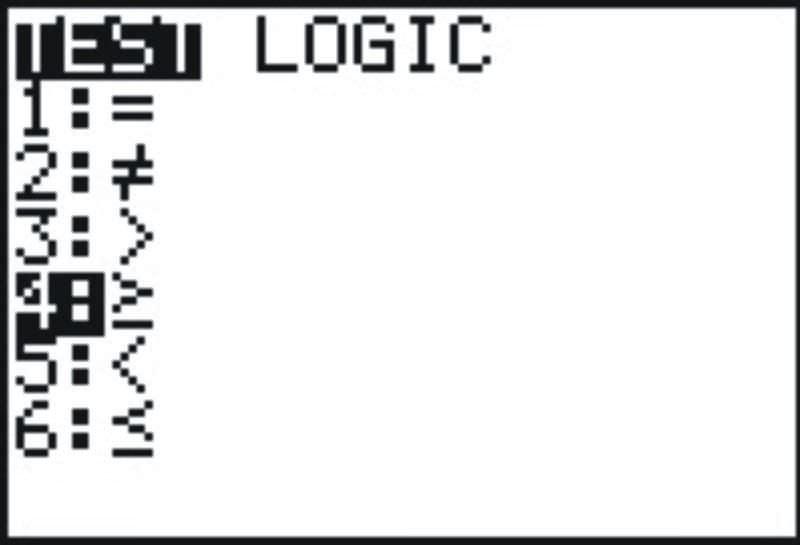Y1=(7x−2<10x+1) AND (10x+1<9x+5)

To enter the [AND] symbol, press [TEST]. Choose [LOGIC] on the top row and then select option 1.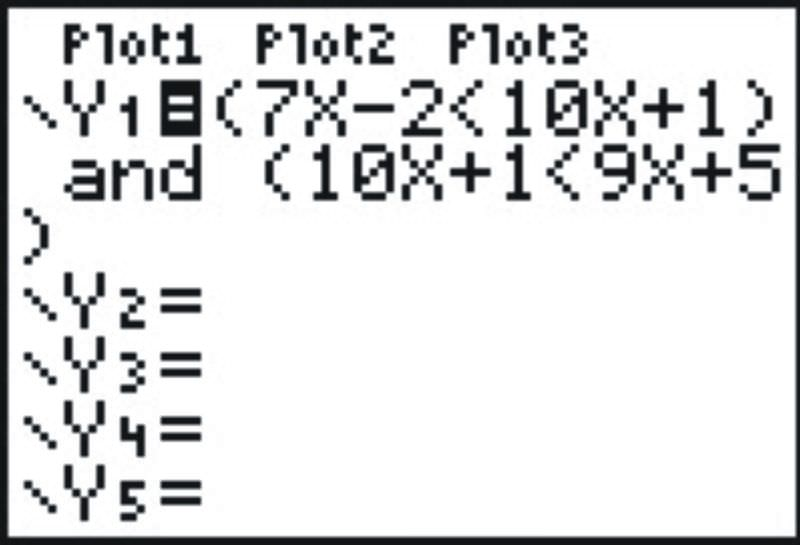The resulting graph is as shown below.

The solutions are the values of x for which y=1.

In this case, −1<x<4 .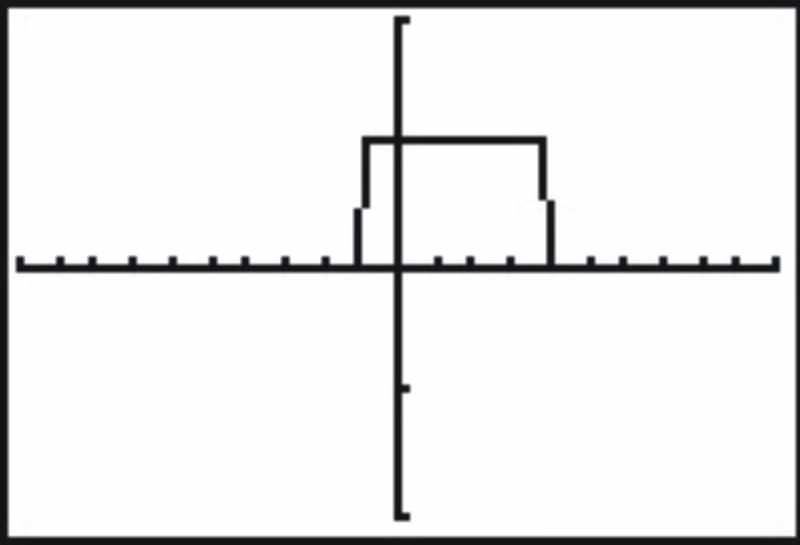### Examples

Example 3.9.1

Earlier, you were told to assume that you spent 2 more hours doing your homework this week than you spent last week. If the number of hours that you spent last week is represented by h, and you spent between 6 and 9 hours this week, how many hours did you spend doing your homework last week?

Solution

If you spent h hours doing your homework this last week, and 2 more hours doing your homework this week, you spent h+2 hours working on homework this week. If you spent 6 to 9 hours working this week, then the inequality that represents this situation is:

6<h+2<9

To solve this, begin by separating the inequalities:

6<h+2 and h+2<9

4<h and h<7

Putting these two inequalities together, you get 4<h<7 as your solution.

You spent between 4 and 7 hours working on homework last week.

Example 3.9.2

Graph the solution set for −1+b>4 or 2b+7≤11.

Solution

Start by solving for b for each inequality.

Simplify by adding opposites. b>5 2b≤4

Divide if necessary. b≤2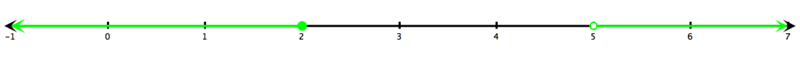### Review

1. Describe the solution set to a compound inequality joined by the word “and.”
2. How would your answer to question #1 change if the joining word was “or?”
3. Write the process used to solve a compound inequality.

Write the compound inequalities represented by the following graphs.

1.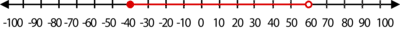2.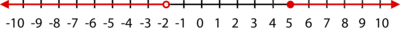3.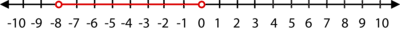4.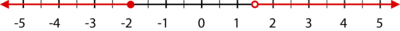5.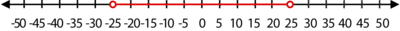6.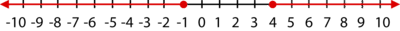7.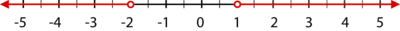Graph each compound inequality on a number line.

1. −4≤x≤6
2. x<0 or x>2
3. x≥−8 or x≤−20
4. −15<x≤85

In 15–30, solve the following compound inequalities and graph the solution on a number line.

1. −5≤x−4≤13
2. −2<4x−5≤11
3. (x−2)/6≤2x−4 or (x−2)/6>x+5
4. 1≤3x+4≤4
5. −12≤2−5x≤7
6. 3/4≤2x+9≤3/2
7. −2<(2x−1)/3<−1
8. 5x+2(x−3)≥2
9. 3x+2≤10 or 3x+2≥15
10. 4x−1≥7 or 9x/2<3
11. 3−x<−4 or 3−x>10
12. (2x+3)/4<2 or (−x/5)+3(2/5)
13. 2x−7≤−3 or 2x−3>11
14. −6d>48 or 10+d>11
15. 6+b<8 or b+6≥6
16. 4x+3≤9 or −5x+4≤−12

### Vocabulary

Term Definition
compound inequalities Inequalities that relate to the same topic can be written as a compound inequality. A compound inequality involves the connecting words and and or.
intersection The word and in mathematics means the intersection between the sets. What the sets have in common.
union The word or in mathematics means the union of the sets.Combining both sets into one large set.

PLIX: Play, Learn, Interact, eXplore: Babysitting

Video:

Activity: Compound Inequalities Discussion Questions

Study Aid: Compound Inequalities Study Guide

Practice: Compound Inequalities

Real World Application: Compound Inequalities